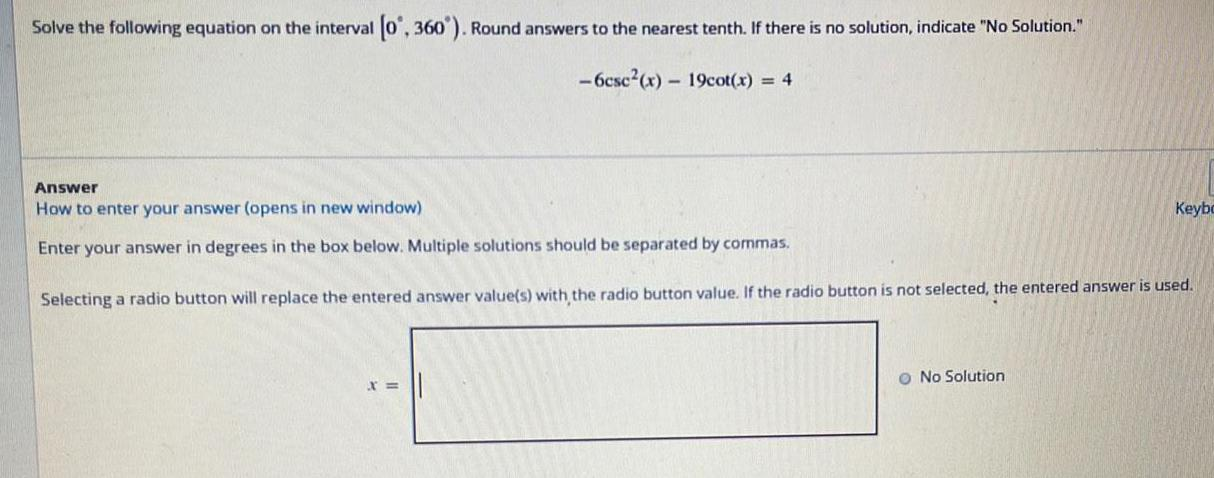Question:

# Solve the following equation on the interval [0°, 360°). Round answers to the nearest tenth. If there is no solution, indicate "No Solution." -6csc²(x) - 19cot(x) = 4 Answer How to enter your answer (opens in new window) Enter your answer in degrees in the

Last updated: 7/28/2022Solve the following equation on the interval [0°, 360°). Round answers to the nearest tenth. If there is no solution, indicate "No Solution." -6csc²(x) - 19cot(x) = 4